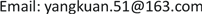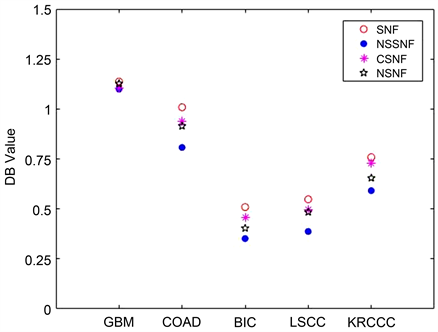﻿ 基于归一化欧氏距离和谱分邻的融合网络对癌症亚型聚类 Clustering of Cancer Subtypes Based on Similarity Network Fusion of Normalized Euclidean Distance and Spectral Neighbor

Pure Mathematics
Vol. 09  No. 10 ( 2019 ), Article ID: 33283 , 8 pages
10.12677/PM.2019.910137

Clustering of Cancer Subtypes Based on Similarity Network Fusion of Normalized Euclidean Distance and Spectral Neighbor

Kuan Yang1, Yaping Zhao2

1School of Mathematical Sciences, Ocean University of China, Qingdao Shandong

2Qingdao Foreign Affairs Service Vocational School, Qingdao ShandongReceived: Nov. 14th, 2019; accepted: Nov. 27th, 2019; published: Dec. 4th, 2019ABSTRACT

Similarity network fusion (SNF) is an effective clustering method to identify cancer subtypes. By using SNF, patient similarity networks for each of their data types are integrated into a single similarity network, which contains all the information of patients. In this paper, a similarity network fusion based on normalized Euclidean distance and spectral clustering (NSSNF) is proposed by using normalized Euclidean distance and redefined neighbor of the patient to reduce the noise of data analysis in similarity networks and increase the complementarity between the data from different similarity networks. Finally, for the five cancer data types from the TCGA database, the data analysis was performed by the NSSNF method, and the results of the evaluation indexes DB and CH showed that the NSSNF method is superior to the SNF method, NSNF method and CSNF method.

Keywords:Subtype, Network Fusion, Normalization, Euclidean Distance, Neighbor, Spectral Clustering, Similarity Network1中国海洋大学数学科学学院，山东 青岛

2青岛外事服务职业学校，山东 青岛1. 引言

2018年4月5日，《Cell》公布了泛癌症图谱(Pan-Cancer Altas)，其分子特征是20,000多种原发性癌症和匹配的33种癌症类型的正常样本，其中，居于癌症发病率首位的肺癌是一种高度错综复杂的异质性疾病，目前肺癌的精确治疗已发展到新的阶段，已确定EGFR突变、ALK融合等七种不同的肺癌亚型，这七种不同的肺癌亚型需要不同的靶向药物进行精确治疗，然而，肺癌以及其它癌症的治疗也存在着许多问题，如何寻找未知的靶基因，确定未知的癌症亚型还需要更多地努力  。相似网络融合是一种有效确定癌症亚型的聚类方法，可以将病人不同的数据类型整合到一个网络中，通过相似网络融合，病人之间的弱相似性减少，降低了数据分析时的噪声，强相似性增加，增加了不同相似网络的数据间的互补性。2014年由Bo Wang  等人首次提出相似网络融合方法((Similarity Network Fusion) SNF)，利用TCGA计划中病人的三种特征DNA甲基化、mRNA表达以及microRNA表达三种数据构建病人的相似融合网络，最后对融合后的网络进行聚类，对比于其它利用单一种数据进行聚类的方法提高了聚类的性能，但也存在着不足，少量的数据样本对应着高维数据，降低数据噪声，扩大数据间的互补性还需要提高。2014年，Jiang Xingpeng  改进了邻居的定义，提出了一致相似网络融合(CSNF)，用一致k近邻代替原来的k近邻方法，以此来推断微生物之间的关系，提高了聚类性能。2017年，Zhang Yong  等人提出一种新的基于相似网络融合的多视图聚类算法(RSNF)，这种方法结合了随机森林的强度优势以及SNF聚类方法的鲁棒性优势，并将其应用于分析人体微生物数据，改善了SNF的聚类性能。2017年，Zhao Yaping  提出了包含邻居信息的相似网络融合(NSNF)，用包含邻居信息的多重紧密k近邻方法代替原来的k近邻方法来构建邻居间的相似网络，将其运用于癌症亚型聚类，与原有方法相比，聚类效果提高很多。2018年，张月  等人，提出了一种基于一致相似度网络融合的极化SAR图像非监督地物分类方法，修正了误分像素的类别标签，提高了地物分类的精度，和传统极化SAR图像地物分类方法相比，聚类性能有了很大的提高。2018年，Ning Chen  提出了基于利用上下文信息改进SNF的信息检索(CI-SNF)，对SNF融合相似性采用Jaccard距离，增强了样本的局部一致性，引入倒排索引技术，提高了聚类的效率，并且将其应用于歌曲识别、图像分类、癌症亚型识别以及药物识别中。

2. 方法

2.1. 数据来源

2.2. 相似网络融合

2.2.1. 构建病人相似网络

$W\left(i,j\right)=\mathrm{exp}\left(-\frac{{d}^{2}\left({x}_{i},{x}_{j}\right)}{\mu {\epsilon }_{i,j}}\right)$(1)

${\epsilon }_{i,j}=\frac{mean\left(d\left({x}_{i},{N}_{i}\right)\right)+mean\left(d\left({x}_{j},{N}_{j}\right)\right)+d\left({x}_{i},{x}_{j}\right)}{3}$(2)

$d\left({x}_{i},{x}_{j}\right)=\frac{\rho \left({x}_{i},{x}_{j}\right)}{\frac{1}{n-1}{\sum }_{l\ne i}^{n}\rho \left({x}_{i},{x}_{l}\right)}$(3)

$\rho \left({x}_{i},{x}_{j}\right)$ 表示病人 ${x}_{i}$${x}_{j}$ 之间的欧氏距离， $mean\left(d\left({x}_{i},{N}_{i}\right)\right)$ 表示距病人 ${x}_{i}$ 最近的k个邻居归一化欧氏距离的平均值，Ni是指包含病人 ${x}_{i}$ 在内距它归一化欧氏距离最近的k个病人组成的邻居。

2.2.2. 病人相似网络标准化

$P\left(i,j\right)=\left\{\begin{array}{l}\frac{W\left(i,j\right)}{{\sum }_{j\ne l}W\left(i,l\right)+{\sum }_{i\ne h}W\left(j,h\right)},\text{\hspace{0.17em}}\text{\hspace{0.17em}}\text{\hspace{0.17em}}j\ne i\\ \frac{1}{2},\text{\hspace{0.17em}}\text{\hspace{0.17em}}\text{\hspace{0.17em}}\text{\hspace{0.17em}}\text{\hspace{0.17em}}\text{\hspace{0.17em}}\text{\hspace{0.17em}}\text{\hspace{0.17em}}\text{\hspace{0.17em}}\text{\hspace{0.17em}}\text{\hspace{0.17em}}\text{\hspace{0.17em}}\text{\hspace{0.17em}}\text{\hspace{0.17em}}\text{\hspace{0.17em}}\text{\hspace{0.17em}}\text{\hspace{0.17em}}\text{\hspace{0.17em}}\text{\hspace{0.17em}}\text{\hspace{0.17em}}\text{\hspace{0.17em}}\text{\hspace{0.17em}}\text{\hspace{0.17em}}\text{\hspace{0.17em}}\text{ }\text{ }\text{\hspace{0.17em}}\text{\hspace{0.17em}}\text{\hspace{0.17em}}\text{\hspace{0.17em}}\text{\hspace{0.17em}}\text{\hspace{0.17em}}\text{\hspace{0.17em}}\text{\hspace{0.17em}}\text{\hspace{0.17em}}\text{\hspace{0.17em}}\text{\hspace{0.17em}}\text{\hspace{0.17em}}\text{\hspace{0.17em}}j=i\end{array}$ (4)

2.2.3. 构建邻居间的相似网络

1) 矩阵W作为相似性矩阵。

2) 求度矩阵D，其中 $D\left(i,j\right)=\left\{\begin{array}{l}{\sum }_{j=1}W\left(i,j\right),\text{\hspace{0.17em}}\text{\hspace{0.17em}}\text{\hspace{0.17em}}i=j\\ 0,\text{\hspace{0.17em}}\text{\hspace{0.17em}}\text{\hspace{0.17em}}\text{\hspace{0.17em}}\text{\hspace{0.17em}}\text{\hspace{0.17em}}\text{\hspace{0.17em}}\text{\hspace{0.17em}}\text{\hspace{0.17em}}\text{\hspace{0.17em}}\text{\hspace{0.17em}}\text{\hspace{0.17em}}\text{\hspace{0.17em}}\text{\hspace{0.17em}}\text{\hspace{0.17em}}\text{\hspace{0.17em}}\text{\hspace{0.17em}}\text{\hspace{0.17em}}i\ne j\end{array}$

3) 求拉普拉斯矩阵 $L=I-{D}^{-1/2}W{D}^{-1/2}$

4) 求L最小的k个特征值对应的特征向量V。

5) 对特征向量V进行K-means聚类。

$S\left(i,j\right)=\left\{\begin{array}{l}\frac{2W\left(i,j\right)}{{\sum }_{l\in {U}_{r}}W\left(i,l\right)+{\sum }_{h\in {U}_{r}}W\left(j,h\right)},\text{\hspace{0.17em}}\text{\hspace{0.17em}}\text{\hspace{0.17em}}\text{\hspace{0.17em}}i,j\in {U}_{r}\\ 0,\text{\hspace{0.17em}}\text{\hspace{0.17em}}\text{\hspace{0.17em}}\text{\hspace{0.17em}}\text{\hspace{0.17em}}\text{\hspace{0.17em}}\text{\hspace{0.17em}}\text{\hspace{0.17em}}\text{\hspace{0.17em}}\text{\hspace{0.17em}}\text{\hspace{0.17em}}\text{\hspace{0.17em}}\text{\hspace{0.17em}}\text{\hspace{0.17em}}\text{\hspace{0.17em}}\text{\hspace{0.17em}}\text{\hspace{0.17em}}\text{\hspace{0.17em}}\text{\hspace{0.17em}}\text{\hspace{0.17em}}\text{\hspace{0.17em}}\text{\hspace{0.17em}}\text{\hspace{0.17em}}\text{\hspace{0.17em}}\text{\hspace{0.17em}}\text{\hspace{0.17em}}\text{\hspace{0.17em}}\text{\hspace{0.17em}}\text{\hspace{0.17em}}\text{\hspace{0.17em}}\text{\hspace{0.17em}}\text{\hspace{0.17em}}\text{\hspace{0.17em}}\text{\hspace{0.17em}}\text{\hspace{0.17em}}\text{\hspace{0.17em}}\text{\hspace{0.17em}}\text{\hspace{0.17em}}\text{\hspace{0.17em}}\text{\hspace{0.17em}}\text{\hspace{0.17em}}\text{otherwise}\end{array}$ (5)

2.2.4. 相似网络融合

${P}_{t+1}^{\left(1\right)}={S}^{\left(1\right)}\frac{{P}_{t}^{\left(2\right)}+{P}_{t}^{\left(3\right)}}{2}{\left({S}^{\left(1\right)}\right)}^{\text{T}}$(6)

${P}_{t+1}^{\left(2\right)}={S}^{\left(2\right)}\frac{{P}_{t}^{\left(1\right)}+{P}_{t}^{\left(3\right)}}{2}{\left({S}^{\left(2\right)}\right)}^{\text{T}}$(7)

${P}_{t+1}^{\left(3\right)}={S}^{\left(3\right)}\frac{{P}_{t}^{\left(1\right)}+{P}_{t}^{\left(2\right)}}{2}{\left({S}^{\left(3\right)}\right)}^{\text{T}}$(8)

$P=\frac{{P}_{t}^{\left(1\right)}+{P}_{t}^{\left(2\right)}+{P}_{t}^{\left(3\right)}}{3}$(9)

2.2.5. 对融合网络进行谱聚类

1) 融合矩阵P作为相似性矩阵。

2) 求度矩阵D，矩阵D是相似矩阵P的度矩阵。

3) 求拉普拉斯矩阵L，其中 $L=I-{D}^{-1/2}P{D}^{-1/2}$

4) 求出L的最小的k个特征值和对应的特征向量V。

5) 将特征向量V进行K-means聚类。

3. 结果

$\text{DB}=\frac{1}{k}{\sum }_{i=1}^{k}\underset{i=1,2,\cdots ,j;i\ne j}{\mathrm{max}}\left\{\frac{\left(\sqrt{\frac{1}{{n}_{i}}{\sum }_{x\in {C}_{i}}{d}^{2}\left(x,{z}_{i}\right)}+\sqrt{\frac{1}{{n}_{j}}{\sum }_{x\in {C}_{j}}{d}^{2}\left(x,{z}_{j}\right)}\right)}{d\left({z}_{i},{z}_{j}\right)}\right\}$(10)

$\text{CH}=\frac{{\sum }_{i=1}^{k}{n}_{i}\cdot {d}^{2}\left({z}_{i},{z}_{tot}\right)}{k-1}\cdot \frac{n-k}{{\sum }_{i=1}^{k}{\sum }_{x\in {C}_{i}}{d}^{2}\left(x,{z}_{i}\right)}$(11)Figure 1. Comparison of CH values of five cancers dataFigure 2. Comparison of DB values of five cancers data

4. 结论

Clustering of Cancer Subtypes Based on Similarity Network Fusion of Normalized Euclidean Distance and Spectral Neighbor[J]. 理论数学, 2019, 09(10): 1115-1122. https://doi.org/10.12677/PM.2019.910137

1. 1. Wu, D., Wang, D.C., Cheng, Y., et al. (2016) Roles of Tumor Heterogeneity in the Development of Drug Resistance: A Call for Precision Therapy. Seminars in Cancer Biology, 42, 13-19. https://doi.org/10.1016/j.semcancer.2016.11.006

2. 2. 周彩存, 刘桑田. 肺癌的靶向治疗与精准医学[J]. 医学研究生学报, 2017, 30(11): 1132-1139.

3. 3. Wang, B., Mezlini, A., Demir, F., et al. (2014) Similarity Network Fusion for Aggregating Data Types on a Genomic Scale. Nature Methods, 11, 333-337. https://doi.org/10.1038/nmeth.2810

4. 4. Jiang, X.P. and Hu, X.H. (2014) Inferring Microbial Interaction Net-works Based on Consensus Similarity Network Fusion. College of Computing and Informatics, 57, 1115-1120. https://doi.org/10.1007/s11427-014-4735-x

5. 5. Zhang, Y., Hua, X. and Jiang, X.P. (2017) Multi-View Cluster-ing of Microbiome Samples by Robust Similarity Network Fusion and Spectral Clustering. IEEE/ACM Transactions on Computational Biology and Bioinformatics, 14, 264-271. https://doi.org/10.1109/TCBB.2015.2474387

6. 6. Zhao, Y.P., Zhao, X.Q., Wang, K.D., et al. (2017) Clustering Cancer Subtypes Based on Neighborhood-Information Embedded Similarity Network Fusion. Periodical of Ocean University of China, A1, 155-160.

7. 7. 张月, 邹焕新, 邵宁远, 等. 基于相似度网络融合的极化SAR图像地物分类[J]. 系统工程与电子技术, 2018, 40(2): 295-302.

8. 8. Chen, N. (2019) CI-SNF: Exploiting Contextual Information to Improve SNF Based Information Retrieval. Information Fusion, 52, 175-186. https://doi.org/10.1016/j.inffus.2018.08.004

9. 9. Qin, D.F., Wengert, C., van Gool, L., et al. (2013) Query Adaptive Similarity for Scale Object Retrieval. Proceedings of the IEEE Conference on Computer Vision and Pattern Recognition, Portland, 23-28 June 2013, 1610-1617.

10. 10. Von Luxburg, U. (2007) A Tutorial on Spectral Clustering. Statistics and Computing, 17, 395-416. https://doi.org/10.1007/s11222-007-9033-z

11. 11. Nigro, J.M., et al. (2005) Integrated Array-Comparative Genomic Hybridization and Expression Array Profiles Identify Clinically Relevant Molecular Subtypes of Glioblastoma. Cancer Research, 65, 1678-1686. https://doi.org/10.1158/0008-5472.CAN-04-2921

12. 12. Davies, D.L. and Bouldin, D.W. (1979) A Cluster Separation Measure. IEEE Transactions on Pattern Analysis Machine Intelligence, 1, 224. https://doi.org/10.1109/TPAMI.1979.4766909

13. 13. Caliski, T. and Harabasz, J. (1974) A Dendrite Method for Cluster Analysis. Communications in Statistics, 3, 1-27. https://doi.org/10.1080/03610917408548446